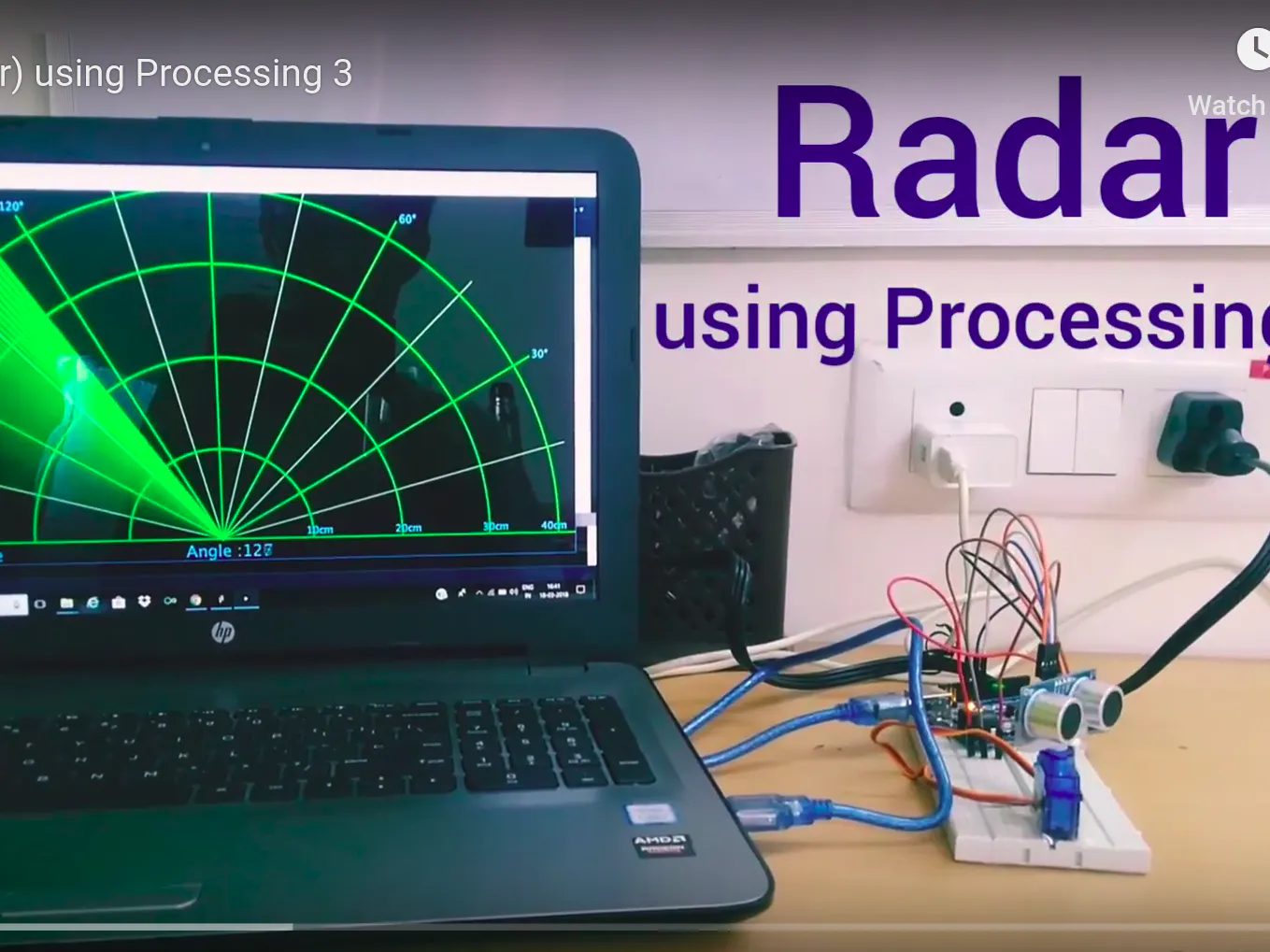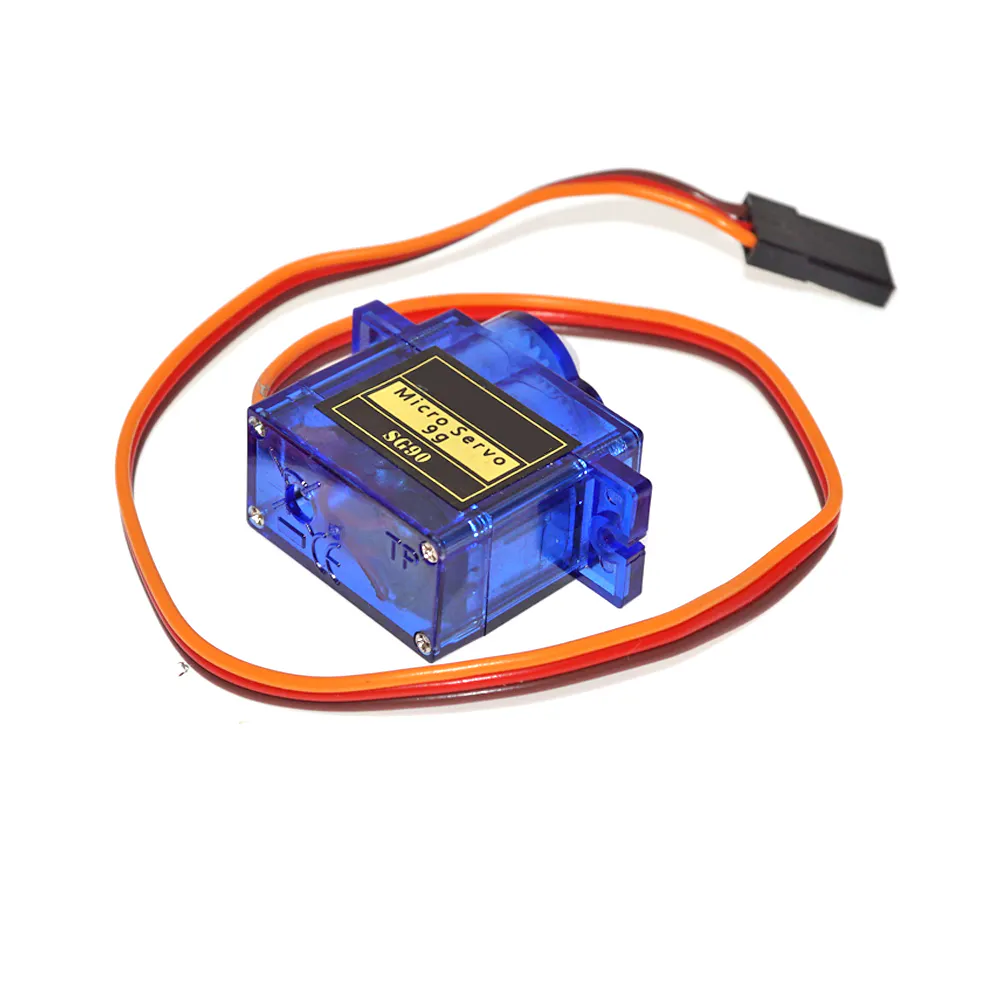Published

# Radar (SONAR) Using Processing 3

We are using an ultrasound sensor that emits a signal and takes a reading which is displayed on screen using Processing 3.

IntermediateFull instructions provided1 hour6,998## Things used in this project

### Hardware componentsArduino UNO & Genuino UNO
×1×1Jumper wires (generic)
×1Ultrasonic Sensor - HC-SR04 (Generic)
×1SG90 Micro-servo motor
×1

## Code

### Arduino IDE Code

C/C++
```#include <Servo.h>

const int trigPin=12;
const int echoPin=11;

long duration;
int distance;

Servo s1;

void setup() {

Serial.begin(9600);
pinMode(trigPin,OUTPUT);
pinMode(echoPin,INPUT);

s1.attach(9);
}

void loop()
{
for(int i=15;i<=165;i++){            // rotates the servo motor from 15 to 165 degrees
s1.write(i);
delay(30);
distance = calDist();

Serial.print(i);                      // Sends the current degree into the Serial Port
Serial.print(",");                   // Sends addition character right next to the previous value needed later in the Processing IDE for indexing
Serial.print(distance);                // Sends the distance value into the Serial Port
Serial.print(".");                   // Sends addition character right next to the previous value needed later in the Processing IDE for indexing
}

for(int i=165;i>15;i--){
s1.write(i);
delay(30);
distance = calDist();
Serial.print(i);
Serial.print(",");
Serial.print(distance);
Serial.print(".");
}

}

int calDist()
{
digitalWrite(trigPin, LOW);
delayMicroseconds(2);
// Sets the trigPin on HIGH state for 10 micro seconds
digitalWrite(trigPin, HIGH);
delayMicroseconds(10);
digitalWrite(trigPin, LOW);
// Reads the echoPin, returns the sound wave travel time in microseconds
duration = pulseIn(echoPin, HIGH);
// Calculating the distance
distance= duration*0.034/2;                                // Range : 2cm to 400 cm

return distance;
}
```

### Processing 3 Code

C/C++
```import processing.serial.*;               // imports library for serial communication

Serial myPort;                         // defines Object for Serial

String ang="";
String distance="";
String data="";

int angle, dist;

void setup() {

size (1200, 700);

myPort = new Serial(this,"COM4", 9600);                     // starts the serial communication
myPort.bufferUntil('.');    // reads the data from the serial port up to the character '.' before calling serialEvent

background(0);
}

void draw() {

//for the blur effect
fill(0,5);              //colour,opacity
noStroke();
rect(0, 0, width, height*0.93);

noStroke();
fill(0,255);
rect(0,height*0.93,width,height);                   // so that the text having angle and distance doesnt blur out

drawLine();
drawObject();
drawText();
}

void serialEvent (Serial myPort) {                                                     // starts reading data from the Serial Port
// reads the data from the Serial Port up to the character '.' and puts it into the String variable "data".
data = data.substring(0,data.length()-1);

int index1 = data.indexOf(",");
ang= data.substring(0, index1);
distance= data.substring(index1+1, data.length());

angle = int(ang);
dist = int(distance);
System.out.println(angle);
}

{
pushMatrix();
noFill();
stroke(10,255,10);        //green
strokeWeight(3);

translate(width/2,height-height*0.06);

line(-width/2,0,width/2,0);

arc(0,0,(width*0.5),(width*0.5),PI,TWO_PI);
arc(0,0,(width*0.25),(width*0.25),PI,TWO_PI);
arc(0,0,(width*0.75),(width*0.75),PI,TWO_PI);
arc(0,0,(width*0.95),(width*0.95),PI,TWO_PI);

stroke(175,255,175);
strokeWeight(1);

popMatrix();
}

void drawLine() {

pushMatrix();

strokeWeight(9);
stroke(0,255,0);
translate(width/2,height-height*0.06);

popMatrix();

}

void drawObject() {

pushMatrix();

strokeWeight(9);
stroke(255,0,0);
translate(width/2,height-height*0.06);

float pixleDist = (dist/40.0)*(width/2.0);                        // covers the distance from the sensor from cm to pixels
float pd=(width/2)-pixleDist;

if(dist<=40)                                                  // limiting the range to 40 cms
{
//line(0,0,pixleDist,0);
}
popMatrix();
}

void drawText()
{
pushMatrix();

fill(100,200,255);
textSize(25);

text("10cm",(width/2)+(width*0.115),height*0.93);
text("20cm",(width/2)+(width*0.24),height*0.93);
text("30cm",(width/2)+(width*0.365),height*0.93);
text("40cm",(width/2)+(width*0.45),height*0.93);

textSize(40);
text("YAinnoware",width*0.08,height*0.99);
text("Angle :"+angle,width*0.45,height*0.99);

if(dist<=40) {
text("Distance :"+dist,width*0.7,height*0.99);
}

translate(width/2,height-height*0.06);
textSize(25);